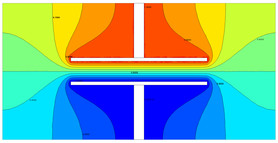# Application Galerie

#### Computing Capacitance

Application ID: 12689

A capacitor, in its simplest form, is a two terminal electrical device that stores electric energy when a voltage difference is applied across the terminals. The stored electric energy is proportional to the applied voltage squared and is quantified by the capacitance of the device. This model introduces a model of a simple capacitor, the electric field and device capacitance are solved for under electrostatic conditions.This model example illustrates applications of this type that would nominally be built using the following products: